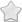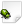CLOSED  Crash report #1403  -  [C7563571] Thread::entryPoint() basic_string.h:2290
Posted Nov 11, 2019 - updated Aug 20, 2021   Shortlink: http://arx.vg/1403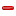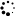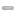0 Votes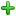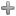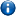This issue is a duplicate of issue #1094 - "[F4AE7D16] Thread::entryPoint() Thread.cpp:144"This issue has been closed with status "Not a bug" and resolution "DUPLICATE".
Issue details
• Type of issue
Crash report
• Status
 Not a bug
• Assigned to
Not assigned to anyone
• Type of bug
Not triaged
• Likelihood
Not triaged
• Effect
Not triaged
• Posted by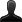CrashBot
• Owned by
Not owned by anyone
• Estimated time
Not estimated
• Category
Not determined
• Resolution
DUPLICATE
• Priority
Not determined
• Reproducability
Not determined
• Severity
Not determined
• Targetted for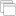Not determined
• OS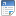Linux
• ArchitectureOther
• Fixed inNot determined
Issue description
Illegal storage access: address not mapped to object

``` Instruction address: 0xfb504
Memory accessed: 0xffffffff81000014
Stack pointer: 0xa2efee38
Frame pointer: 0xa2efef7c
```

Callstack:
``` arx!0xf5870
??!0xb6808130
arx!0xfb504
```

GDB stack trace:
``` [New LWP 23881]
[New LWP 23882]
[New LWP 23883]
[New LWP 23884]
0xb6f73afc in memcpy () from /usr/lib/arm-linux-gnueabihf/libarmmem-v7l.so
Id   Target Id                                   Frame
* 1    Thread 0xb6faf010 (LWP 23874) "arx"         0xb6f73afc in memcpy () from /usr/lib/arm-linux-gnueabihf/libarmmem-v7l.so
2    Thread 0xb4eb5440 (LWP 23881) "threaded-ml" __GI___poll (timeout=90, nfds=3, fds=0xaff05798) at ../sysdeps/unix/sysv/linux/poll.c:29
3    Thread 0xa3eff440 (LWP 23882) "arx"         futex_wait_cancelable (private=0, expected=0, futex_word=0xaaeac8) at ../sysdeps/unix/sysv/linux/futex-internal.h:88
4    Thread 0xa32ff440 (LWP 23883) "arx"         0xb6f1c088 in futex_abstimed_wait_cancelable (private=0, abstime=0x0, expected=1, futex_word=0xab1514) at ../sysdeps/unix/sysv/linux/futex-internal.h:205
5    Thread 0xa2eff440 (LWP 23884) "arx"         __GI___nanosleep (remaining=0x0, requested_time=0xa2efea94) at ../sysdeps/unix/sysv/linux/nanosleep.c:28

#0  __GI___nanosleep (remaining=0x0, requested_time=0xa2efea94) at ../sysdeps/unix/sysv/linux/nanosleep.c:28
_a1 = -1561335148
_nr = 162
_a1tmp = -1561335148
_a2tmp = 0
_a2 = 0
_sys_result = <optimized out>
sc_cancel_oldtype = 0
sc_ret = <optimized out>
sc_ret = <optimized out>
sc_ret = <optimized out>
_sys_result = <optimized out>
_a1 = <optimized out>
_nr = <optimized out>
_a2tmp = <optimized out>
_a1tmp = <optimized out>
_a2 = <optimized out>
sc_cancel_oldtype = <optimized out>
_sys_result = <optimized out>
_a1 = <optimized out>
_nr = <optimized out>
_a2tmp = <optimized out>
_a1tmp = <optimized out>
_a2 = <optimized out>
#1  __GI___nanosleep (requested_time=0xa2efea94, remaining=0x0) at ../sysdeps/unix/sysv/linux/nanosleep.c:25
sc_ret = <optimized out>
_sys_result = <optimized out>
_a1 = <optimized out>
_nr = <optimized out>
_a2tmp = <optimized out>
_a1tmp = <optimized out>
_a2 = <optimized out>
sc_cancel_oldtype = <optimized out>
_sys_result = <optimized out>
_a1 = <optimized out>
_nr = <optimized out>
_a2tmp = <optimized out>
_a1tmp = <optimized out>
_a2 = <optimized out>
#2  0x000f58d4 in CrashHandlerPOSIX::handleCrash (context=0xa2efeb40, info=0xa2efeac0, signal=<optimized out>, this=0x7793e8) at /media/pi/Linux/ArxLibertatis/src/platform/crashhandler/CrashHandlerPOSIX.cpp:500
t = {tv_sec = 0, tv_nsec = 100000}
processor = 23885
args = {0x0, 0x186a0 <__static_initialization_and_destruction_0(int, int) [clone .constprop.382] 37200> "\003", 0x0}
processor = <optimized out>
args = <optimized out>
it = <optimized out>
siginfo = <optimized out>
ctx = <optimized out>
t = <optimized out>
#3  signalHandler (signal=<optimized out>, info=0xa2efeac0, context=0xa2efeb40) at /media/pi/Linux/ArxLibertatis/src/platform/crashhandler/CrashHandlerPOSIX.cpp:84
No locals.
#4  <signal handler called>
No locals.
#5  0x000fb504 in Thread::entryPoint (param=0x81000010) at /usr/include/c  /8/bits/basic_string.h:2290
ret = <optimized out>
start = <optimized out>
pd = 0xa2eff440
unwind_buf = {cancel_jmp_buf = {{jmp_buf = {-275174083, -74979539, -1225033368, -1561332672, -1225067312, 338, -1093850126, -1561332672, 0, -1561333892, 0 <repeats 54 times>}, mask_was_saved = 0}}, priv = {pad = {0x0, 0x0, 0x0, 0x0}, data = {prev = 0x0, cleanup = 0x0, canceltype = 0}}}
not_first_call = <optimized out>
#7  0xb68b2578 in ?? () at ../sysdeps/unix/sysv/linux/arm/clone.S:73 from /lib/arm-linux-gnueabihf/libc.so.6
No locals.
Backtrace stopped: previous frame identical to this frame (corrupt stack?)

#0  0xb6f1c088 in futex_abstimed_wait_cancelable (private=0, abstime=0x0, expected=1, futex_word=0xab1514) at ../sysdeps/unix/sysv/linux/futex-internal.h:205
_a2tmp = 393
_a2 = 393
_v2 = -1
_a3tmp = 1
_a3 = 1
_a1 = 11212052
_nr = 240
_a4tmp = 0
_a1tmp = 11212052
_a4 = 0
_v1 = 0
__ret = <optimized out>
oldtype = 0
err = <optimized out>
oldtype = <optimized out>
err = <optimized out>
__ret = <optimized out>
_a1 = <optimized out>
_nr = <optimized out>
_v2tmp = <optimized out>
_v1tmp = <optimized out>
_a4tmp = <optimized out>
_a3tmp = <optimized out>
_a2tmp = <optimized out>
_a1tmp = <optimized out>
_a2 = <optimized out>
_a3 = <optimized out>
_a4 = <optimized out>
_v1 = <optimized out>
_v2 = <optimized out>
#1  do_futex_wait (sem=sem@entry=0xab1514, abstime=0x0) at sem_waitcommon.c:115
err = <optimized out>
#2  0xb6f1c1f4 in __new_sem_wait_slow (sem=0xab1514, abstime=0x0) at sem_waitcommon.c:282
_buffer = {__routine = 0xb6f1c050 <__sem_wait_cleanup>, __arg = 0xab1514, __canceltype = -1009807360, __prev = 0x0}
err = 0
v = <optimized out>
#3  0xb6dac7b0 in ?? () from /usr/lib/arm-linux-gnueabihf/libopenal.so.1
No symbol table info available.
Backtrace stopped: previous frame identical to this frame (corrupt stack?)

#0  futex_wait_cancelable (private=0, expected=0, futex_word=0xaaeac8) at ../sysdeps/unix/sysv/linux/futex-internal.h:88
_a1 = 11201224
_nr = 240
_a3tmp = 0
_a1tmp = 11201224
_a3 = 0
_a4tmp = 0
_a2tmp = 128
_a2 = 128
_a4 = 0
__ret = <optimized out>
oldtype = 0
err = <optimized out>
oldtype = <optimized out>
err = <optimized out>
__ret = <optimized out>
_a1 = <optimized out>
_nr = <optimized out>
_a4tmp = <optimized out>
_a3tmp = <optimized out>
_a2tmp = <optimized out>
_a1tmp = <optimized out>
_a2 = <optimized out>
_a3 = <optimized out>
_a4 = <optimized out>
spin = 0
buffer = {__routine = 0xb6f18e28 <__condvar_cleanup_waiting>, __arg = 0xa3efed98, __canceltype = 0, __prev = 0x0}
cbuffer = {wseq = 24, cond = 0xaaeaa0, mutex = 0xaff05168, private = 0}
__atg101_val = <optimized out>
rt = <optimized out>
__atg100_val = <optimized out>
err = <optimized out>
g = 0
flags = <optimized out>
g1_start = <optimized out>
signals = <optimized out>
result = 0
wseq = 103079215104
seq = 11758924396879348952
private = 0
maxspin = <optimized out>
err = <optimized out>
result = <optimized out>
wseq = <optimized out>
g = <optimized out>
seq = <optimized out>
flags = <optimized out>
private = <optimized out>
signals = <optimized out>
g1_start = <optimized out>
__atg6_oldval = <optimized out>
__atg6_memp = <optimized out>
__atg6_value = <optimized out>
__atg2_result = <optimized out>
__oldval = <optimized out>
__atg101_val = <optimized out>
__atg100_val = <optimized out>
spin = <optimized out>
buffer = <optimized out>
cbuffer = <optimized out>
__atg101_val = <optimized out>
__atg100_val = <optimized out>
__atg6_oldval = <optimized out>
__atg6_memp = <optimized out>
__atg6_value = <optimized out>
__atg2_result = <optimized out>
__oldval = <optimized out>
__atg101_val = <optimized out>
__atg100_val = <optimized out>
rt = <optimized out>
__atg101_val = <optimized out>
__atg100_val = <optimized out>
__atg102_expected = <optimized out>
__atg1_result = <optimized out>
__oldval = <optimized out>
s = <optimized out>
__atg100_val = <optimized out>
__atg102_expected = <optimized out>
__atg1_result = <optimized out>
__oldval = <optimized out>
No locals.
#3  0xb4694084 in pa_threaded_mainloop_wait () from /usr/lib/arm-linux-gnueabihf/libpulse.so.0
No symbol table info available.
#4  0xb6da4c68 in ?? () from /usr/lib/arm-linux-gnueabihf/libopenal.so.1
No symbol table info available.
Backtrace stopped: previous frame identical to this frame (corrupt stack?)

#0  __GI___poll (timeout=90, nfds=3, fds=0xaff05798) at ../sysdeps/unix/sysv/linux/poll.c:29
_a1 = -1343203432
_nr = 168
_a3tmp = 90
_a1tmp = -1343203432
_a3 = 90
_a2tmp = 3
_a2 = 3
_sys_result = <optimized out>
sc_cancel_oldtype = 0
sc_ret = <optimized out>
sc_ret = <optimized out>
sc_ret = <optimized out>
_sys_result = <optimized out>
_a1 = <optimized out>
_nr = <optimized out>
_a3tmp = <optimized out>
_a2tmp = <optimized out>
_a1tmp = <optimized out>
_a2 = <optimized out>
_a3 = <optimized out>
sc_cancel_oldtype = <optimized out>
_sys_result = <optimized out>
_a1 = <optimized out>
_nr = <optimized out>
_a3tmp = <optimized out>
_a2tmp = <optimized out>
_a1tmp = <optimized out>
_a2 = <optimized out>
_a3 = <optimized out>
#1  __GI___poll (fds=0xaff05798, nfds=3, timeout=90) at ../sysdeps/unix/sysv/linux/poll.c:26
sc_ret = <optimized out>
_sys_result = <optimized out>
_a1 = <optimized out>
_nr = <optimized out>
_a3tmp = <optimized out>
_a2tmp = <optimized out>
_a1tmp = <optimized out>
_a2 = <optimized out>
_a3 = <optimized out>
sc_cancel_oldtype = <optimized out>
_sys_result = <optimized out>
_a1 = <optimized out>
_nr = <optimized out>
_a3tmp = <optimized out>
_a2tmp = <optimized out>
_a1tmp = <optimized out>
_a2 = <optimized out>
_a3 = <optimized out>
#2  0xb4693968 in ?? () from /usr/lib/arm-linux-gnueabihf/libpulse.so.0
No symbol table info available.
Backtrace stopped: previous frame identical to this frame (corrupt stack?)

#0  0xb6f73afc in memcpy () from /usr/lib/arm-linux-gnueabihf/libarmmem-v7l.so
No symbol table info available.
#1  0x00000000 in ?? ()
No symbol table info available.
Backtrace stopped: previous frame identical to this frame (corrupt stack?)
[Inferior 1 (process 23874) detached]

```

Steps to reproduce this issue
Hi I am trying to make it run on rpi4. I can use 2.1 gl desktop profile or 3.1 ES gl right now, 3.2 ES its comming on next gen mesa v3d drivers... but i dont think than this crash its related buy any means with that. my email its salva.liebana@gmail.com

#3
Comment posted byCrashBot
Nov 11, 04:22
A file was uploaded. cfg.ini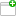This comment was attached:

arxcrash-23874-1409346823
#1
Comment posted byCrashBot
Nov 11, 04:22
A file was uploaded. arx.logThis comment was attached:

arxcrash-23874-1409346823
#4
Comment posted byCrashBot
Nov 11, 04:22
A file was uploaded. maps.txtThis comment was attached:

arxcrash-23874-1409346823
#5
Comment posted byCrashBot
Nov 11, 04:22
A file was uploaded. crash.txtThis comment was attached:

arxcrash-23874-1409346823
#6
Comment posted byDaniel Scharrer
Dec 10, 03:44
ARM isn't expected to work yet. Not sure what is going wrong here though.